### Home > A2C > Chapter 12 > Lesson 12.5.1 > Problem12-199

12-199.
1. You know that the solutions of the general quadratic equation ax2 + bx + c = 0 are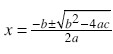. Homework Help ✎

1. Find the sum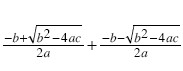.

2. Find the product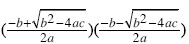.

3. How is the sum of the solutions related to the original quadratic equation?

4. How is the product of the solutions related to the original quadratic equation?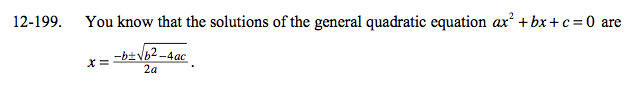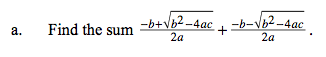$-\frac{b}{a}$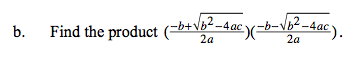$\frac{c}{a}$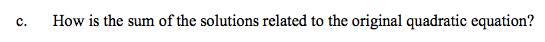What are a and b in the original equation?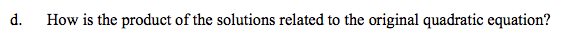What are a and c in the original equation?